# Analytic function, element of an

(diff) ← Older revision | Latest revision (diff) | Newer revision → (diff)

The collection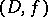of domainsin the plane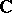of a complex variableand analytic functions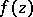given onby a certain analytic apparatus that allows one to effectively realize the analytic continuation ofto its whole domain of existence as a complete analytic function. The simplest and most frequently used form of an element of an analytic function is the circular element in the form of a power series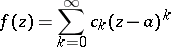(1)

and its disc of convergence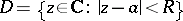with centre at(the centre of the element) and radius of convergence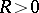. The analytic continuation here is achieved by a (possibly repeated) re-expansion of the series (1) for various centres,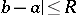, by formulas like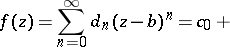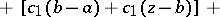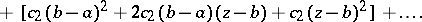Any one of the elementsof a complete analytic function determines it uniquely and can be represented by means of circular elements with centres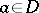. In the case of the centre at infinity,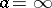, the circular element takes the form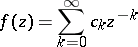with domain of convergence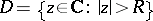.

In the process of the analytic continuation,may turn out to be multiple-valued and there may appear corresponding algebraic branch points (cf. Algebraic branch point), that is, branched elements of the form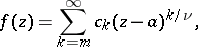where; the number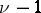is called the branching order. The branched elements generalize the concept of an element of an analytic function, which in this connection is also called an unramified (for) regular (for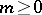) element.

As the simplest elementof an analytic functionof several complex variables,, one can take a multiple power series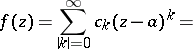(2)where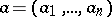is the centre,,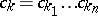,, andis some polydisc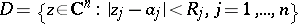in which the series (2) converges absolutely. However, forone has to bear in mind that a polydisc is not the exact domain of absolute convergence of a power series.

The concept of an element of an analytic function is close to that of the germ of an analytic function.

How to Cite This Entry:
Analytic function, element of an. Encyclopedia of Mathematics. URL: http://encyclopediaofmath.org/index.php?title=Analytic_function,_element_of_an&oldid=14787
This article was adapted from an original article by E.D. Solomentsev (originator), which appeared in Encyclopedia of Mathematics - ISBN 1402006098. See original article